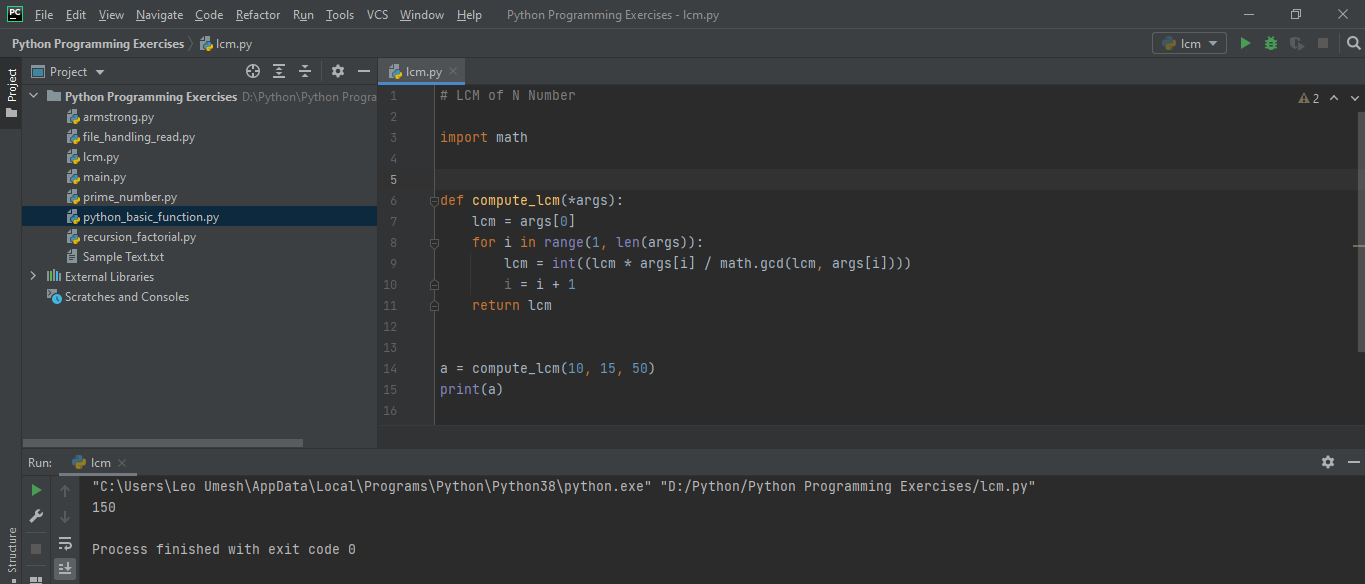# PYTHON PROGRAMMING EXERCISES 4: FINDING LCM OF N NUMBERS

in STEMGeeks5 months ago

The following is a simple python program to calculate the LCM of any given numbers. You can find Lowest Common Multiple (LCM) of two numbers or three numbers or four numbers. I was looking in google to find a simple way to find the lcm of number but all of them had a long code and somewhat difficult logic to find the lcm. So, I decided to find the lcm using my own way in python using key-worded argument function.

``````import math

def compute_lcm(*args):
lcm = args
for i in range(1, len(args)):
lcm = int((lcm * args[i] / math.gcd(lcm, args[i])))
i = i + 1
return lcm

a = compute_lcm(10, 15, 50)
print(a)
``````

Running this code will give you following output:This code can be modified in many ways like you can pass different argument to `compute_lcm` function. You can use a loop asking user to enter the number for finding lcm. Its super easy to do this task with python. I had used python inbuilt function called `gcd()`, to find the lcm of the number. This inbuilt function only takes two parameter.